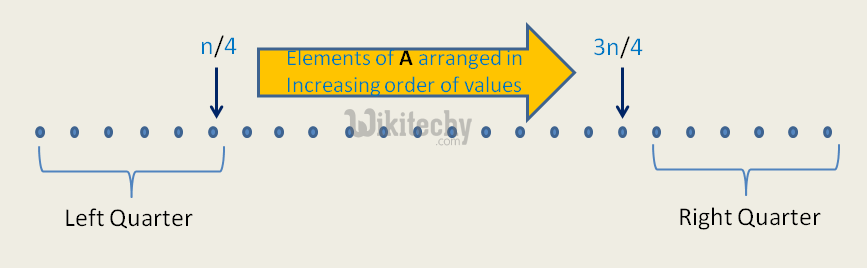Java Programming – Randomized Algorithms (1/2) Approximate Median

It is quite easy to visualize this statement since the median which we report will be (k/2)th element and if we take k/2 elements from the left quarter.

Problem Statement : Given an unsorted array A[] of n numbers and ε > 0, compute an element whose rank (position in sorted A[]) is in the range [(1 – ε)n/2, (1 + ε)n/2].
For ½ Approximate Median Algorithm &epsilom; is 1/2 => rank should be in the range [n/4, 3n/4]

We can find k’th smallest element in O(n) expected time and O(n) worst case time.

What if we want in less than O(n) time with low probable error allowed?
Following steps represent an algorithm that is O((Log n) x (Log Log n)) time and produces incorrect result with probability less than or equal to 2/n2.
Randomly choose k elements from the array where k=c log n (c is some constant)
Insert then into a set.
Sort elements of the set.
Return median of the set i.e. (k/2)th element from the set.

Java Program
/* Java program to find Approximate Median using
1/2 Approximate Algorithm */
import java.util.Iterator;
import java.util.Random;
import java.util.TreeSet;

class Test
{
static int arr[] = new int[]{1, 3, 2, 4, 5, 6, 8, 7} ;

// This function returns the Approximate Median
static int randApproxMedian(int n)
{
// Declaration for the random number
Random r = new Random();

if (n==0)
return 0;

double k1 = 10*Math.log(n); // Taking c as 10

int k = (int)k1;

// A treeset stores unique elements in sorted order
TreeSet s = new TreeSet<Integer>();
for (int i=0; i<k; i++)
{
// Generating a random index
// Random number generated will be in the range [0,n-1]
int index = r.nextInt(n);

//Inserting into the set
}

Iterator<Integer> itr = s.iterator();

int temp = s.size()/2 - 1;

for (int i = 0; i < temp; i++) {
itr.next();
}

// Return the median
return itr.next();
}

// Driver method to test the above function
public static void main(String[] args) {

System.out.println("Approximate Median is " + randApproxMedian(arr.length));

}
}

Time Complexity:
We use a set provided by the STL in C++. In STL Set, insertion for each element takes O(log k). So for k insertions, time taken is O (k log k).
Now replacing k with c log n
=>O(c log n (log (clog n))) =>O (log n (log log n))

How is probability of error less than 2/n2?
Algorithm makes an error if the set S has at least k/2 elements are from the Left Quarter or Right Quarter.

READ  Java Programming - Length of the largest subarray with contiguous elementsIt is quite easy to visualize this statement since the median which we report will be (k/2)th element and if we take k/2 elements from the left quarter(or right quarter) the median will be from the left quarter (or the right quarter).

An array can be divided into 4 quarters each of size n/4. So P(selecting left quarter) is 1/4. So what is the probability that at least k/2 elements are from the Left Quarter or Right Quarter? This probability problem is same as below :

Given a coin which gives HEADS with probability 1/4 and TAILS with 3/4. The coin is tossed k times. What is the probability that we get at least k/2 HEADS is less than or equal to?

Explanation:If we put k = c log n for c = 10, we get
P <= (1/2)2log n
P <= (1/2)log n2
P <= n-2

Probability of selecting at least k/2 elements from the left quarter) <= 1/n2
Probability of selecting at least k/2 elements from the left or right quarter) <= 2/n2

Therefore algorithm produces incorrect result with probability less that or equal to 2/n2.